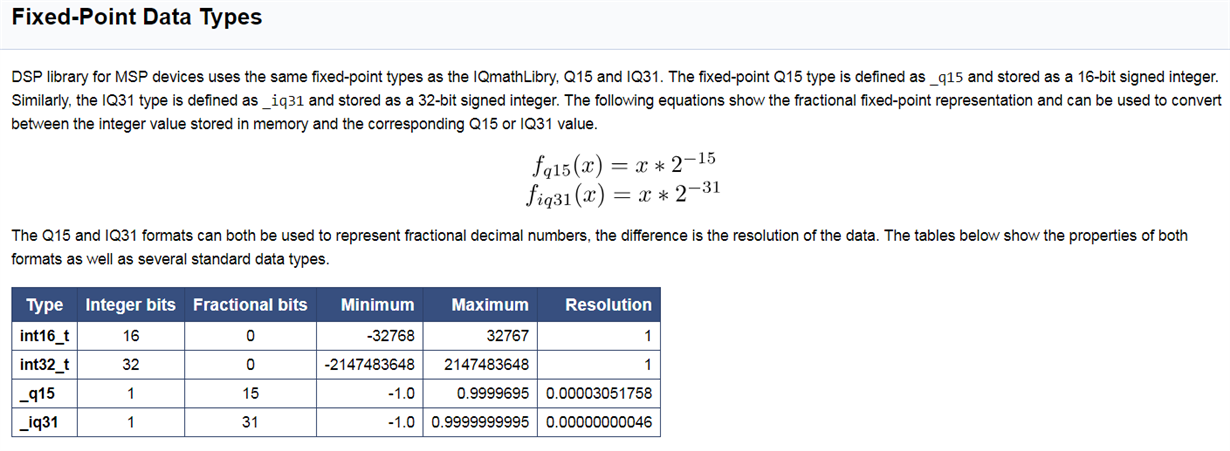If you have a related question, please click the "Ask a related question" button in the top right corner. The newly created question will be automatically linked to this question.

# MSP430FR5992: MSP-DSPLib & msp_matrix_mpy_q15

Part Number: MSP430FR5992
Other Parts Discussed in Thread: MSP-DSPLIB,

Dear team.

My customer uses MSP430FR5992 and  MSP-DSPLib. He wants to do Matrix multiplication.

So he uses matrix_ex3_mpy_q15.c(DSPLib_1_30_00_02\examples\Matrix\matrix_ex3_mpy_q15) and msp_matrix_mpy_q15. But he got different result when he tested the msp_matrix_mpy_q15.

example:

inputA=1132     2132      132     1132     132     132

inputB=199       299        33        44         55        66

1 when use MSP_USE_LEA

Result       213  242   25   29

2  when NOT use MSP_USE_LEA  and define  __MSP430_HAS_MPY32__

Result       9   13   7   10

MSP_USE_LEA   and NOT define __MSP430_HAS_MPY32__

Result       9   13   7   10

Coulld anyone explain this?

BR,

Susan Yang

• Hi Susan Yang,

The function: msp_matrix_mpy_q15 in DSP library has two options use or NOT use LEA and use or NOT use MPY32. But the MPY32 will not be available if the LEA is used.

So is the third state you listed above is wrong? It must be "define MSP_USE_LEA and NOT define __MSP430_HAS_MPY32__"?

B.R.

Longyu Fang

• Hi Susan,

The msp_matrix_mpy_q15 function requires that all rows and columns must be a multiple of two (http://software-dl.ti.com/msp430/msp430_public_sw/mcu/msp430/DSPLib/latest/exports/html/structmsp__matrix__mpy__q15__params.html). By changing the sizes to [2x4] * [4x2] and padding with zeros I get the same results of 9, 13, 7, 10 using LEA. See the modified example code below.

Generally LEA is best suited when doing large vector or matrix operations. When the vector length or matrix size is large padding with zeros does not have a big impact of computation time (e.g. rounding vector length of 127 up to 128 is less than 1% increase). When the vector or matrix is small padding with zeros will have a larger impact on the cycle count (e.g. [2x4] * [4x2] is 33% more than [2x3] * [3x2]). For small operations LEA may or may not be faster and more energy efficient than using the HW multiplier because of the overhead when using LEA (generally takes 50-60 cycles to setup and invoke).

Regards,

Brent Peterson

```#include "msp430.h"

#include <stdint.h>
#include <stdbool.h>

#include "DSPLib.h"

/* Input signal parameters */
#define SIGNAL_ROWS1        2
#define SIGNAL_COLS1        4
#define SIGNAL_ROWS2        4
#define SIGNAL_COLS2        2

/* Input matrix A */
DSPLIB_DATA(inputA,4)
_q15 inputA[SIGNAL_ROWS1][SIGNAL_COLS1] = {
{1132,   2132,   132,    0},
{1132,   132,    132,    0}
};

/* Input matrix B */
DSPLIB_DATA(inputB,4)
_q15 inputB[SIGNAL_ROWS2][SIGNAL_COLS2] = {
{199,   299},
{33,    44},
{55,    66},
{0,     0}
};

/* Result of the matrix add */
DSPLIB_DATA(result,4)
_q15 result[SIGNAL_ROWS1][SIGNAL_COLS2];

/* Benchmark cycle counts */
volatile uint32_t cycleCount;

void main(void)
{
msp_status status;
msp_matrix_mpy_q15_params mpyParams;

/* Disable WDT. */
WDTCTL = WDTPW + WDTHOLD;

#ifdef __MSP430_HAS_PMM__
/* Disable GPIO power-on default high-impedance mode for FRAM devices */
PM5CTL0 &= ~LOCKLPM5;
#endif

/* Initialize the parameter structure. */
mpyParams.srcARows = SIGNAL_ROWS1;
mpyParams.srcACols = SIGNAL_COLS1;
mpyParams.srcBRows = SIGNAL_ROWS2;
mpyParams.srcBCols = SIGNAL_COLS2;

/* Invoke the msp_matrix_mpy_q15 API. */
msp_benchmarkStart(MSP_BENCHMARK_BASE, 1);
status = msp_matrix_mpy_q15(&mpyParams, *inputA, *inputB, *result);
cycleCount = msp_benchmarkStop(MSP_BENCHMARK_BASE);
msp_checkStatus(status);

/* End of program. */
__no_operation();
}
```

• 3.  NOT define  MSP_USE_LEA   and NOT define __MSP430_HAS_MPY32__

• It turns out that after I modify the matrix, the result of settlement is 9, 13, 7, 10..
InputA = {{
{1132, 2132, 132, 0},
{1132, 132, 132, 0}
};
InputB = {{
{199, 299},
{33, 44},
{55, 66},
{0, 0}
};

The result of manual calculation should be:
1132*199+2132*33+132*55+0*0=302884
1132*299+2132*44+132*66+0*0=440988
1132*199+132*33+132*55+0*=236884
132*299+132*44+132*66+0*0=352988

After 15 right shifts:
302884>>15=9
440988>>15=13
236884>>15=7
352988>>15=10

My question is the result of the calculation of the library function. Why do I have to move to the right 15 bits before the result is consistent with the manual calculation?
• Hi,

I think the reason may be that the result in function msp_matrix_mpy_q15 is type _q15, and the inputs are also _q15. The conversion isSo the real calculate process is _q15 * _q15 = REAL DATA A * REAL DATA B * 2^(-15) * 2^(-15).

So the result of the function is REAL DATA A * REAL DATA B * 2^(-15).

And the result is _q15, so the real result is (result of the function) * 2^(-15).

For example,

The real result of 9 = 9 * 2(-15) = 0.00027466.

The real calculate process is (1132*199+2132*33+132*55+0*0) * 2^(-15) * 2^(-15) = 302884 * 2^(-15) * 2^(-15) = 0.00028208.

There may be some errors, but the result is correct.

B.R.

Longyu Fang

• So the matrix multiplication function returns an approximate value? Is it possible to increase the accuracy of the calculation by enlarging the value of the input matrix and reducing the result?
For example:
Input A=input A*1000;
Input B=input B*1000;
Result=result/1000/1000;

•  The inputs of the matrix multiplication function are fixed-point number, if you want to do multiplication of integer, you can modify the source code of the function.

For example,

Change type of result from _q15 to int32_t.

Remove the operation of shift right 15 bits. The specific code is as below:

1. Not use LEA. Not use MPY32
Change
`dst[dst_row_offset + dst_col] = (_q15)__saturate(result >> 15, INT16_MIN, INT16_MAX);`

To

`dst[dst_row_offset + dst_col] = (int32_t)__saturate(result, INT32_MIN, INT32_MAX);`
2. Not use LEA. Use MPY32
Change
`dst[dst_row_offset + dst_col] = RESHI;`

To

`dst[dst_row_offset + dst_col] = RESHI * 32768 + RESLO;`
3. Use LEA